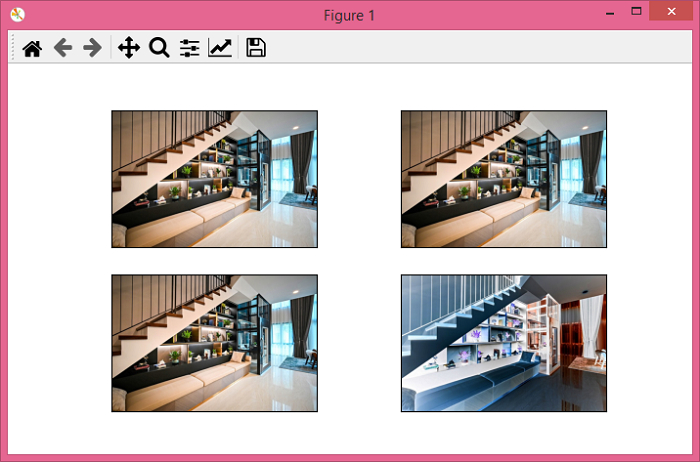# PyTorch â€“ How to invert the colors of an image randomly with a given probability?

The RandomInvert() transform inverts the colors of an image randomly with a given probability. The torchvision.transforms module provides many important transforms that can be used to perform different types of manipulations on the image data.

RandomInvert() accepts both PIL and tensor images or batch of tensor images. A tensor image is a PyTorch Tensor with shape [3, H, W], where H is the image height and W is the image width. A batch of tensor images is also a torch tensor with [B, 3, H, W] where B is the number of images in the batch.

## Syntax

torchvision.transforms.RandomInvert(p)(img)

It returns a randomly color inverted image with a given probability p.

• If p = 1, it returns color inverted image.

• If p = 0, It returns the original image.

• If p is in range (0,1), then the probability to return the randomly color inverted image is p.

• img is input PIL image or tensor image.

## Steps

We could use the following steps to invert colors of the input image randomly with a given probability −

• Import the required libraries. In all the following examples, the required Python libraries are torch, Pillow, and torchvision. Make sure you have already installed them.

import torch
import torchvision
import torchvision.transforms as T
from PIL import Image
• Read the input image. The input image is a PIL image or a torch tensor.

img = Image.open('stairs.jpg')
• Define a transform to invert the colors of the original input image randomly with a given probability p.

transform = T.RandomInvert(p = 0.25)
• Apply the above-defined transform on the input image to invert colors of input image.

inverted_img = transform(img)
• Show the randomly color inverted image.

inverted_img.show()

## Input Image

This image is used as the input file in all the following examples.## Example 1

In this program, we set the probability to 1 so that the output will definitely be a color-inverted image.

# import the required libraries
import torch
import torchvision.transforms as T
from PIL import Image

img = Image.open('stairs.jpg')

# define a transform to randomly invert
# the color with probability=1
transform = T.RandomInvert(p=1)

# apply the above transform on input image
img = transform(img)
img.show()

## Output

It will produce the following output −## Example 2

Let's take another example −

import torch
import torchvision.transforms as T
from PIL import Image
import matplotlib.pyplot as plt

img = Image.open('stairs.jpg')

# define transform with probability = 0.25
transform = T.RandomInvert(p=0.25)

# apply the transform four times
invert_imgs = [transform(img) for _ in range(4)]
fig = plt.figure(figsize=(7,4))
rows, cols = 2,2
for j in range(0, len(invert_imgs)):
plt.imshow(invert_imgs[j])
plt.xticks([])
plt.yticks([])
plt.show()

## Output

It will produce the following output −Notice in the above output, out of the four images, at least one image is color-inverted because we have set the probability as 0.25.